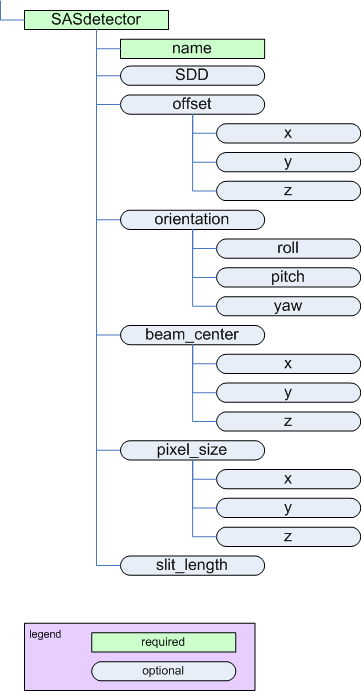SAScollimation

SASprocess

# SASdetector¶

parent:
SASinstrumentThe SASdetector element

Name Type Occurrence Description Attributes
name string [1..1] Identifies the name of this detector.
SDD float [0..1] Distance between sample and detector. unit={unit} 
offset container [0..1] Offset of this detector position in X, Y, (and Z if necessary).
orientation container [0..1] Orientation (rotation) of this detector in roll, pitch, and yaw.
beam_center container [0..1] Center of the beam on the detector in X, Y, (and Z if necessary).
pixel_size container [0..1] Size of detector pixels in X, Y, (and Z if necessary).
slit_length float [0..1] Slit length of the instrument for this detector, expressed in the same units as $$Q$$. (See Rules) unit={unit} 

## geometry¶

See the figures in Definition of the coordinate axes.

## offset¶

Name Type Occurrence Description Attributes
$$x$$ float [0..1] Offset of the detector position in X. unit={unit} 
$$y$$ float [0..1] Offset of the detector position in Y. unit={unit} 
$$z$$ float [0..1] Offset of the detector position in Z. unit={unit}  

## orientation¶

Note

The orientation element is intended to describe simple rotations about a single axis rather than a full set of rotations as in a crystallographic context.

Name Type Occurrence Description Attributes
roll float [0..1] Rotation about the Z axis (roll). unit={unit} 
pitch float [0..1] Rotation about the X axis (pitch). unit={unit} 
yaw float [0..1] Rotation about the Y axis (yaw). unit={unit} 

## beam_center¶

Position of the beam center on the detector

Name Type Occurrence Description Attributes
$$x$$ float [0..1] Position of the beam center on the detector in X. unit={unit} 
$$y$$ float [0..1] Position of the beam center on the detector in Y. unit={unit} 
$$z$$ float [0..1] Position of the beam center on the detector in Z. unit={unit}  

## pixel_size¶

Name Type Occurrence Description Attributes
$$x$$ float [0..1] Size of a detector pixel in X. unit={unit} 
$$y$$ float [0..1] Size of a detector pixel in Y. unit={unit} 
$$z$$ float [0..1] Size of a detector pixel in Z. unit={unit}  

Table Notes

  (1, 2, 3, 4, 5, 6, 7, 8, 9, 10, 11, 12, 13, 14) The unit attribute is required. See Rules for acceptable values.
  (1, 2, 3) While $$z$$ is allowed by the standard (provided by use of a standard size element in the XML Schema), it does not make sense to use it for small-angle scattering in some situations as noted. Use of $$z$$ in such situations may be ignored by processing software.